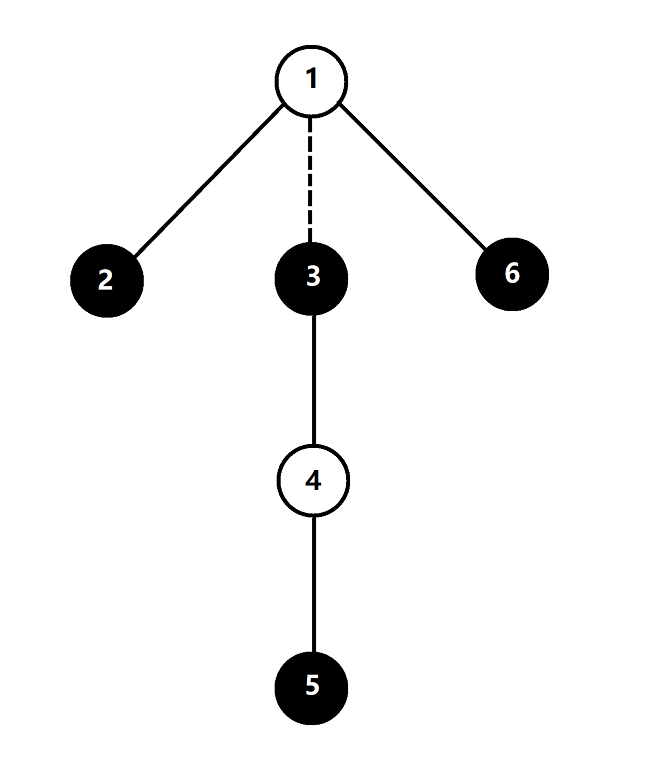# #708. 【UER #10】磁球与磁棍

### 样例一

#### input

1
6 2
1 1 3 4 1



#### output

2



#### explanation### 样例二

#### input

1
3 2
1 1



#### output

-1



### 数据范围与提示

$1$ $15$ $200$ $10$
$2$ $2000$ $2000$ $30$
$3$ $2 \times 10^5$ $2 \times 10^5$ 除点 $1$ 外所有点度数不超过 $2$ $10$
$4$ $2 \times 10^5$ $2 \times 10^5$ $20$
$5$ $10^6$ $10^6$ $30$